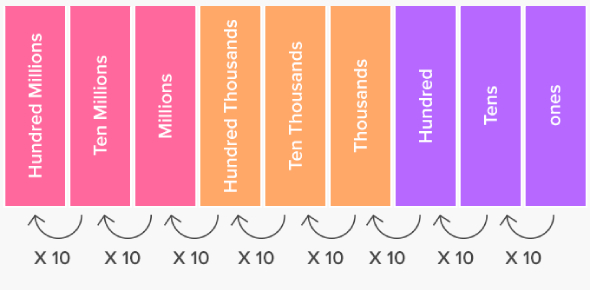# Place Value Quiz Exam MCQ!

14 Questions | Attempts: 5202SettingsThis quiz is developed for elementary students and Math lovers! If you're willing to accept the challenge, then only attempt this quiz. There is a list of 14 questions. Take this quiz to test your mathematical skills and knowledge about place value. Read the questions carefully and take your time.

• 1.
What place value is the underlined digit?  132,864
• A.

Hundreds

• B.

Tens

• C.

Ones

• D.

Thousands

• 2.
What place value is the underlined number?  573,968
• A.

Hundreds

• B.

Ones

• C.

Tens

• D.

Ten Thousands

• 3.
Which symbol goes in the blank?  6,523   ____   9,700
• A.

• B.

>

• C.

=

• 4.
Which symbol means less than?
• A.

• B.

>

• C.

=

• 5.
What is the place value of the underlined number?  539,538
• A.

Ones

• B.

Tens

• C.

Thousands

• D.

Hundred thousands

• 6.
What is the place value of the underlined number?  389,462
• A.

Thousands

• B.

Ten thousands

• C.

Tens

• D.

Hundred thousands

• 7.
What is this number?  500 + 30 +4
• A.

453

• B.

543

• C.

354

• D.

534

• 8.
What is the expanded notation of this number?  918
• A.

900+8 +1

• B.

900+80+10

• C.

900+18

• D.

900+10+8

• 9.
What is this number?  2000 + 700 + 90 + 5
• A.

2,795

• B.

9,270

• C.

5,927

• D.

2,759

• 10.
What is the expanded notation of this number?  9284
• A.

200+80+4+9000

• B.

900+200+80+4

• C.

9000 + 200 + 80 + 4

• D.

2000+ 900+ 8 +40

• 11.
Arrange these digits to make the biggest possible number.  8        1          9
• A.

918

• B.

981

• C.

819

• D.

198

• 12.
Arrange these digits to make the biggest possible number.  4        5       0      6
• A.

5,064

• B.

6,054

• C.

6,540

• D.

4,506

• 13.
Arrange these digits to make the biggest possible number:  195,724
• A.

497,215

• B.

975,421

• C.

125,749

• D.

975,412

• 14.
Write this number in words:  125
• A.

One hundred twenty five

• B.

One hundred fifty two

• C.

Two hundred twenty five

• D.

One hundred and two

## Related TopicsBack to top
×

Wait!
Here's an interesting quiz for you.